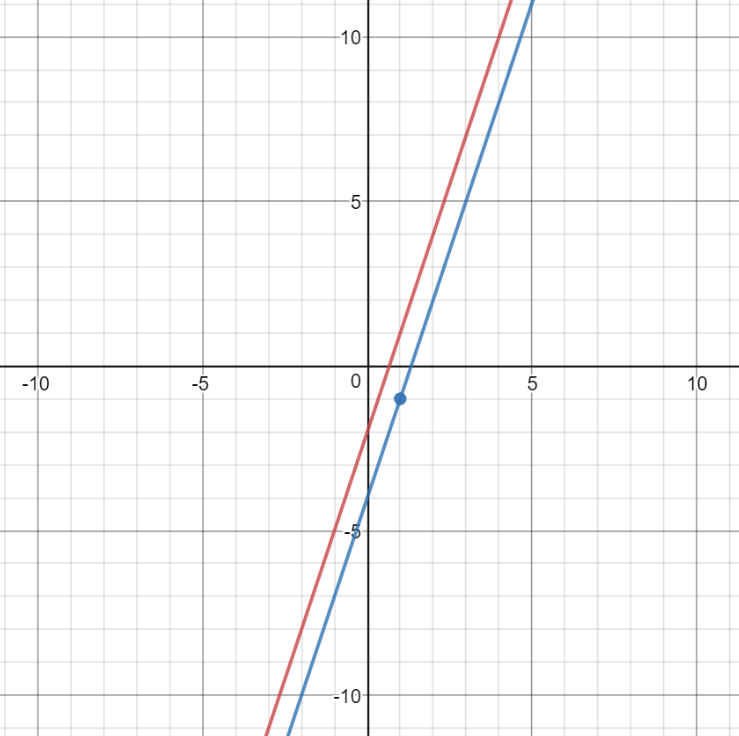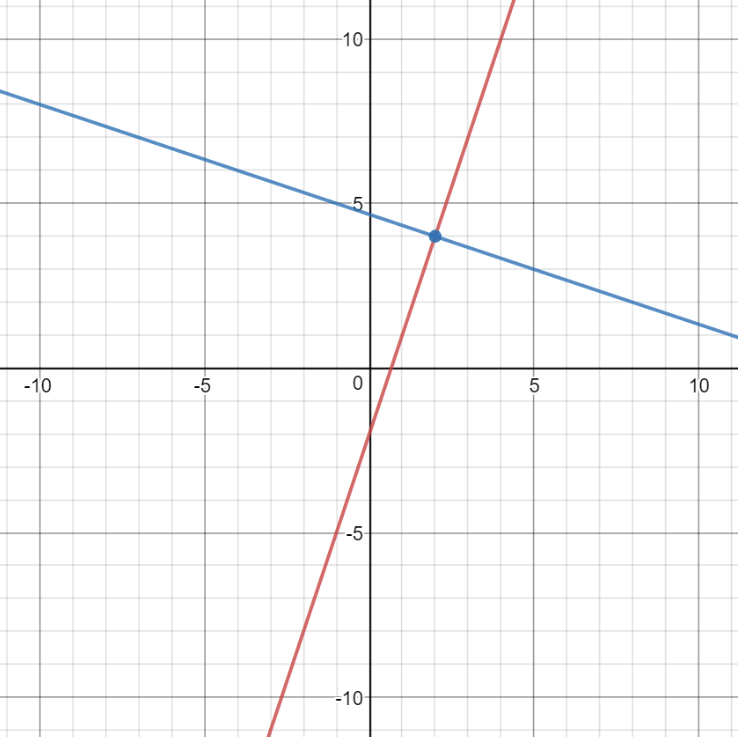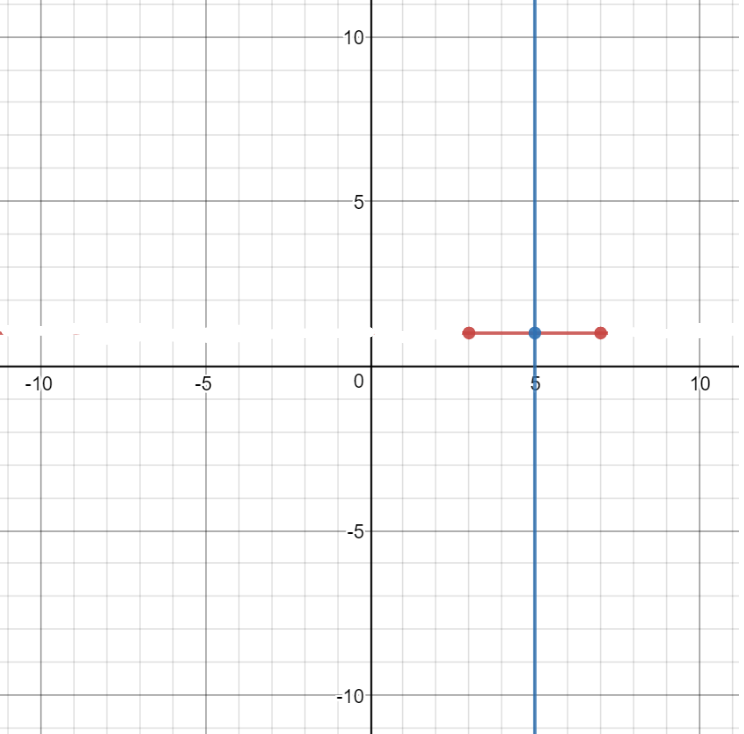# LINR 1 | Lesson 4 | Practice (Writing the Equation of Parallel and Perpendicular Lines) Solutions

1. The slope of the line is $$3$$.  The equation of the line parallel to $$y = 3x-1$$ and through the point $$(1,-1)$$ is $$y = 3x-4$$ in Slope-Intercept Form.1. The slope of the line is $$3$$.  The slope of the line perpendicular to $$y=3x-2$$ and through the point $$(2, 4)$$ is $$-\dfrac{1}{3}$$.  The equation is $$x+3y = 14$$ in Standard Form.1. The midpoint of the line segment is $$(5, 1)$$. The slope of the line segment is 0, and the slope of the perpendicular line is  $$-\dfrac{1}{0}$$ or undefined.  We know this to be a vertical line.  Therefore the equation is $$x = 5$$.1. The midpoint of the segment is $$(3, 5,)$$.  The slope of the segment is $$1$$. The slope of the line perpendicular to the line segment and through the point $$(3,5)$$ is $$-1$$.  The equation of the perpendicular bisector in Standard Form is $$x+y = 8$$.